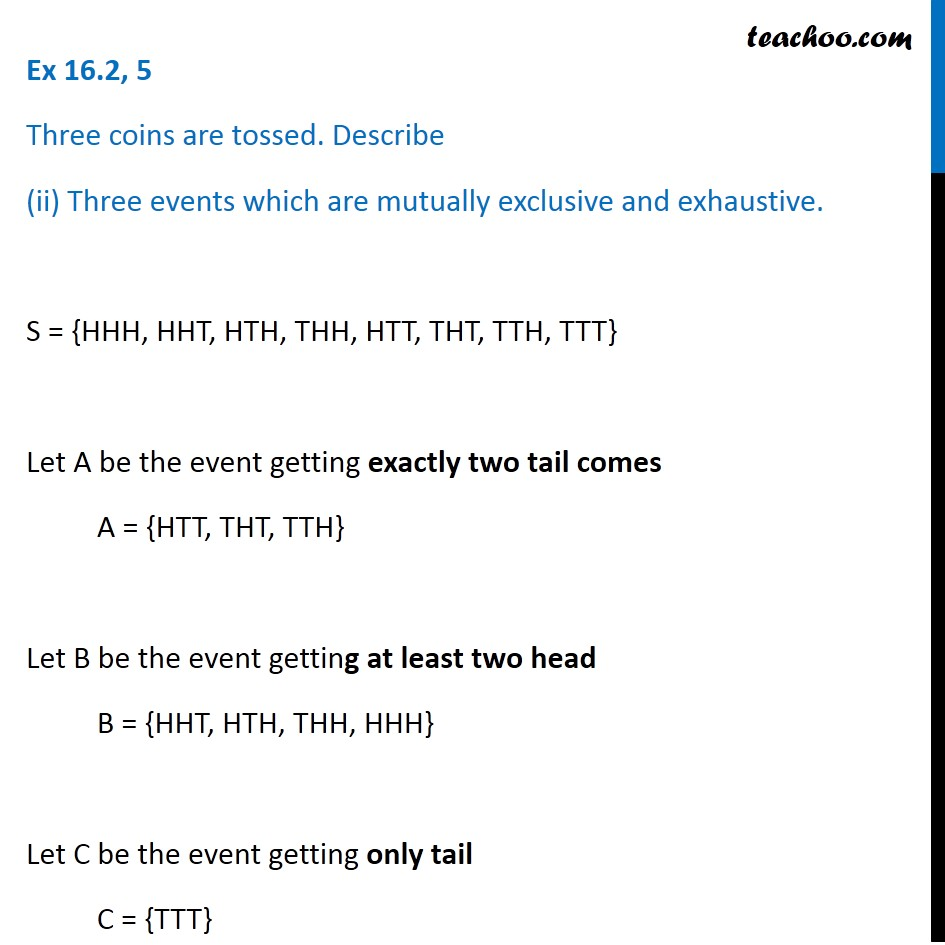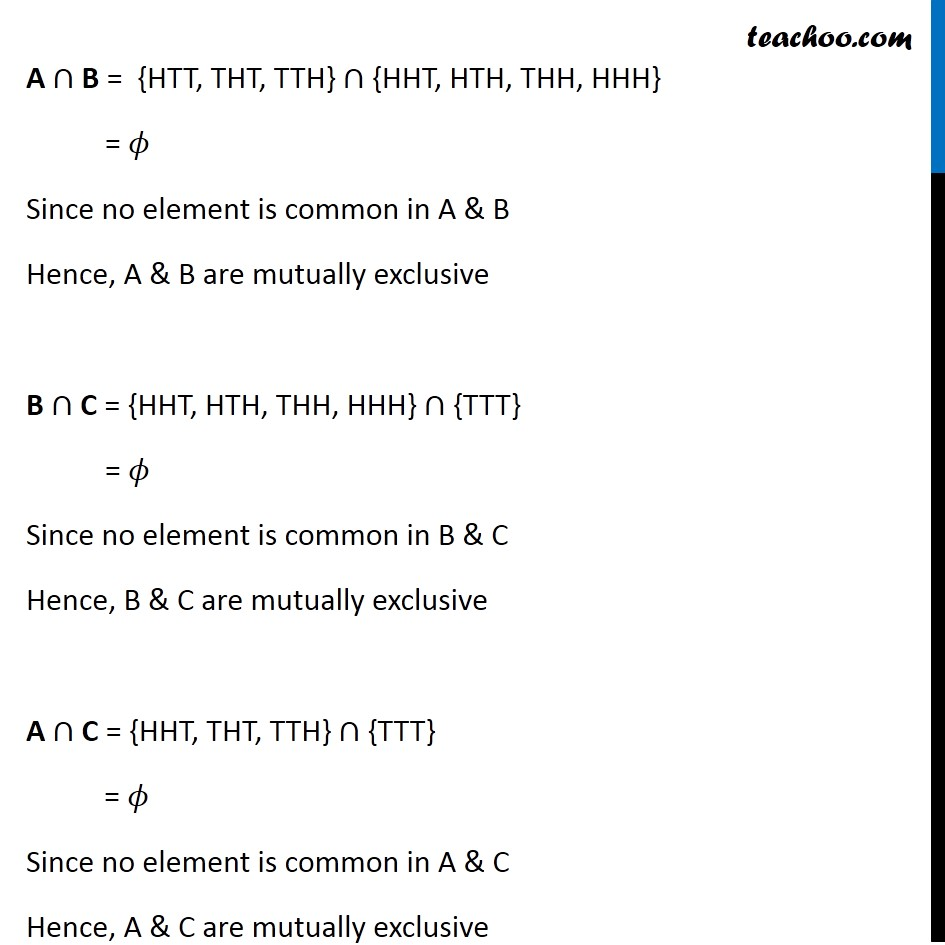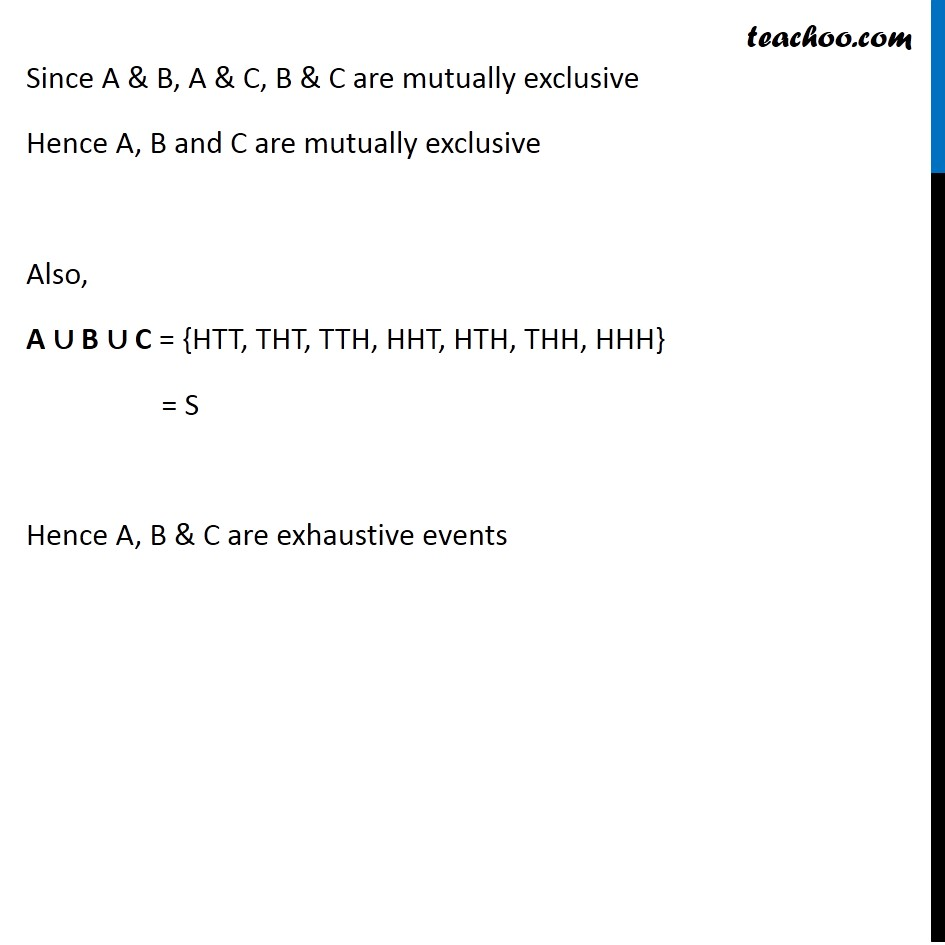Ex 16.2

Chapter 16 Class 11 Probability
Serial order wiseGet live Maths 1-on-1 Classs - Class 6 to 12

### Transcript

Ex 16.2, 5 Three coins are tossed. Describe (ii) Three events which are mutually exclusive and exhaustive. S = {HHH, HHT, HTH, THH, HTT, THT, TTH, TTT} Let A be the event getting exactly two tail comes A = {HTT, THT, TTH} Let B be the event getting at least two head B = {HHT, HTH, THH, HHH} Let C be the event getting only tail C = {TTT} A ∩ B = {HTT, THT, TTH} ∩ {HHT, HTH, THH, HHH} = 𝜙 Since no element is common in A & B Hence, A & B are mutually exclusive B ∩ C = {HHT, HTH, THH, HHH} ∩ {TTT} = 𝜙 Since no element is common in B & C Hence, B & C are mutually exclusive A ∩ C = {HHT, THT, TTH} ∩ {TTT} = 𝜙 Since no element is common in A & C Hence, A & C are mutually exclusive Since A & B, A & C, B & C are mutually exclusive Hence A, B and C are mutually exclusive Also, A ∪ B ∪ C = {HTT, THT, TTH, HHT, HTH, THH, HHH} = S Hence A, B & C are exhaustive events